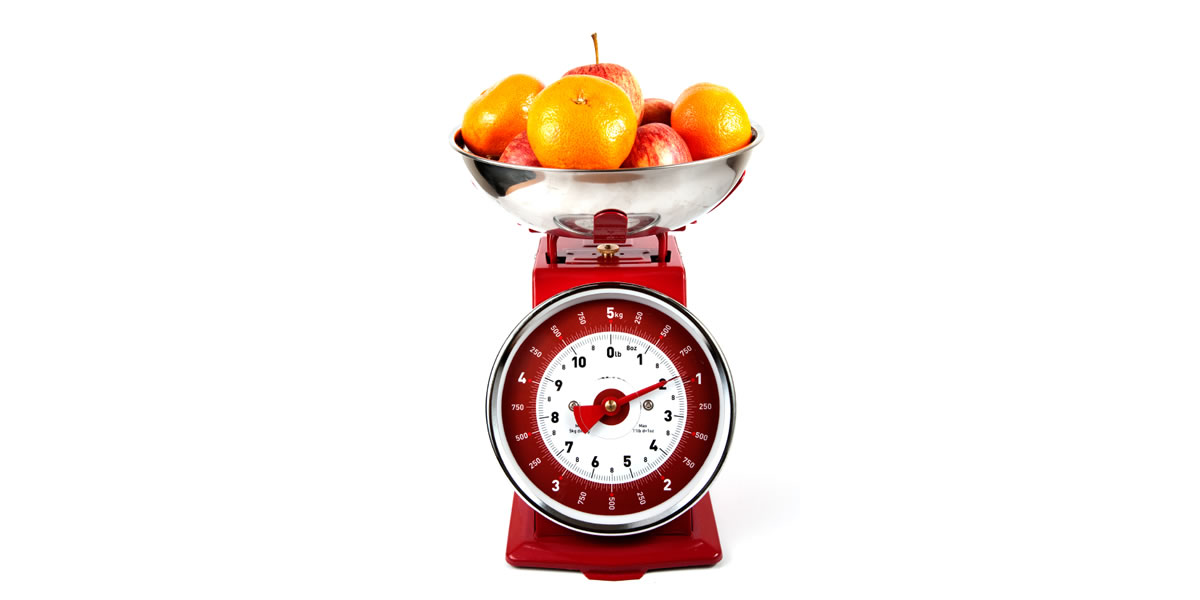# Which BMR formula is most accurate?## Which BMR formula is most accurate?

Hi Jeff, this is an excellent question, and if I would be able to answer it, I would have probably won a prestigious scientific prize. BMR stands for “Basal Metabolic Rate”, which is a measure of how many calories you are using when you are just “being”. More specifically, it measures how many calories you are consuming while in a rested post-absorptive state (your digestive system is inactive after ~12 hours of fasting) in a neutral environment. In this state, it is only the vital processes, such as lungs, heart, etc… which are expending energy.

Generally, BMR is measured for research purposes and for individuals in intensive care with a condition such as cancer or recovering from burns, where it is very important to get an accurate estimate of caloric needs.

Measuring BMR is very difficult, since there are very precise guidelines which must be followed closely. Therefore, it is not usually BMR which is measured, but actually RMR, or “Resting Metabolic Rate”, which is very similar, but with less strict conditions.

BMR/RMR can be calculated by breathing into a device called a calorimeter, which measures the volumes of the different types of gases (O2 and CO2) inhaled and exhaled. From this ratio, it is able to calculate an estimate of caloric expenditure. Since calorimeters are expensive, formulas have been developed, which can be used in their place.

There are several different formulas which are utilized. On this site, we use the Harris-Benedict formula, which is the most widely used one. There are other formulas such as the Katch-Mcardle and the Miffin. Different formulas are more accurate for different people, and if you really want to see which is most accurate, you may have to go to a gym or research center which has a calorimeter which you can use and then compare its reading to the formulas.

I personally don’t think it usually matters much which formula you decide to use since most give results which are very similar. Also, keep in mind that all of these formulas calculate your RMR, which is only ~ 60-70% of your daily caloric expenditure.

Another 20% is due to activity, and the remaining ~10% is due to the thermogenic effect of food, or the energy used for digestion. Most online calculators take this into account and ask for your activity level in order to calculate this into the equation.